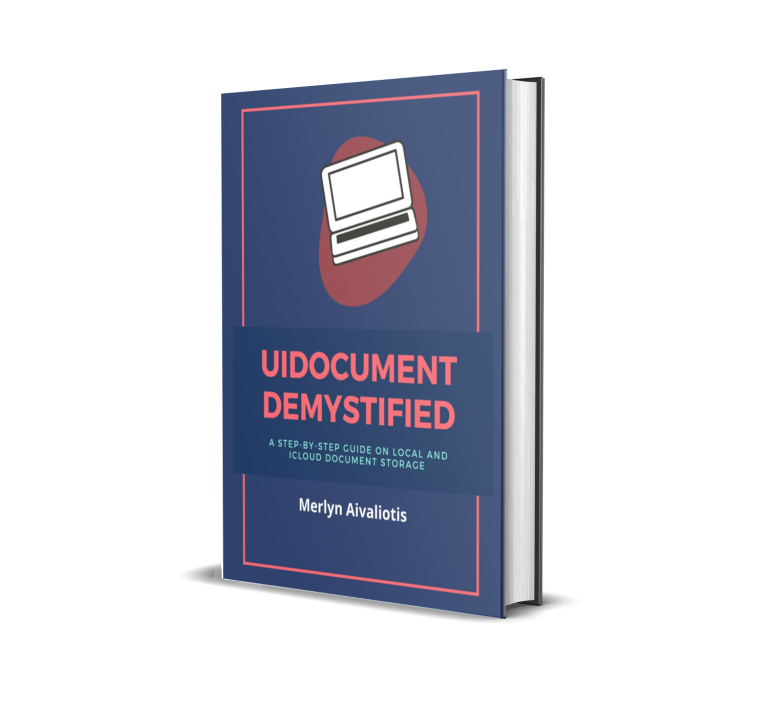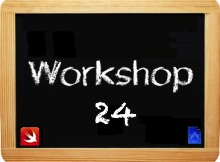A function is a piece of Swift code that can be executed once or many times by an iOS application. In this workshop you learn how to define and use our own functions and how to use them to help write iOS applications.

 Before you begin, I assume you have done the following: Read workshop 1-23 Launched the project, Swift Variable.xcodeproj in Xcode 6 IDE-see image below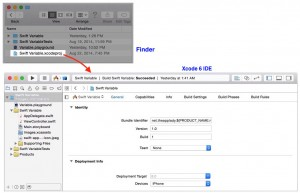#### How Functions Work

This section of the workshop discuss how functions work and you learn how to define and use simple functions.

A function should perform a single, well-defined task. To accomplish this task, the function might require zero or more values, called parameters or arguments, that are pass to it by the calling function. When the function has completed its task, it can return no or one value, called the return value, to the calling function. The calling function can then use the return value in any of its statements. Diagram 1 shows a generalized function.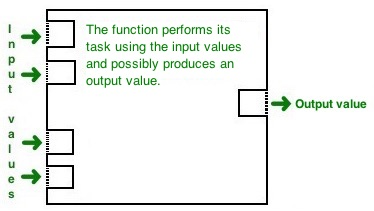Diagram 1: A Generalized Function

For example, suppose that we need to calculate the sales tax on the amount of the purchase in an iOS application. Diagram 2 shows a function called calculateSalesTax() that could perform this task.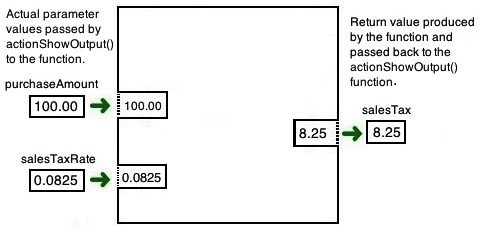Diagram 2: calculateSalesTax() Function

The function calculateSalesTax() requires two parameters because the function must be given the value of the purchase and the applicable sales tax rate. The function actionShowOutput() , which is the calling function in this case, passes the values of purchaseAmount and salesTaxRate to the function. The function uses these values, namely 100.00 and 0.0825 , to calculate the sales tax. The function then passes the value of the sales tax back to actionShowOutput(), which assigns it to the variable salesTax. Exactly how the function computes the sales tax and returns its value is not important right now. You will learn how to do these things later in this workshop.

#### Void Function

A void function is one that require no parameters and return no value. The syntax of this type of function look like this:

Here is an example of how to create a void function. Add it before the closing } in the Swift Variable project’s ViewController.swift class file.

 ```class ViewController: UIViewController, UITextViewDelegate { . . . func sayHello() { let name = "John Smith" outputTextView.text = "\n\nHello, \(name)" } }```

#### Call A Function

To use the sayHello function, you have to call or invoke it in the “Show Output” button’s IBAction method like this:

```@IBAction func actionShowOutput(sender: AnyObject) {
sayHello()
}```

When you run the Swift Variable application in the iOS Simulator and click the Show Output button; you will see this output in the Text View control.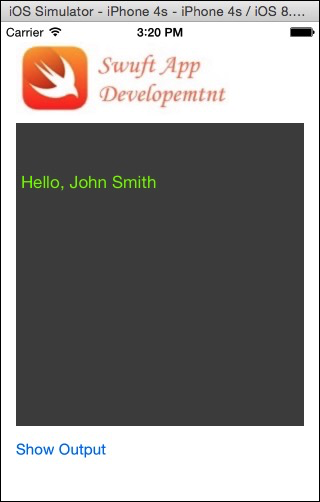#### A Function With Parameters and No Return Value

There’s another kind of function you can create in a Swift class file. It takes one or more arguments and return no value. The values that a function receives from the calling function are stored in the function’s arguments, which are variables enclosed in parenthesis (). The syntax of this kind of function is as follows.

Each data item we pass to a function requires its own argument.

In Diagram 3 below, ordinary variables such as ch, i, and j are represented by closed squares. Function arguments are represented as squares with one side open to the outside of the function box. This suggest that arguments receive their values from outside the function.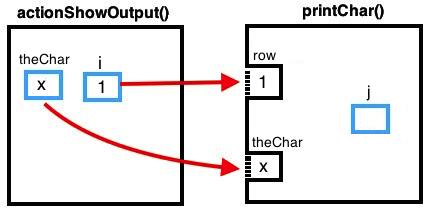Diagram 3: How variables and a function’s arguments are represented

Say we wanted the Swift variable application to display these characters in the Text View control.

We can easily solve this problem using nested for loops. However, instead we will use a function to solve the problem. The app must display five lines. Each line has one more character than the preceding line. We shall us one function, printChar(), to produce each of these lines. Thus, we need to pass information to the function, namely the character (x) we want the app to display and the number of times (5) to display it.

The function printChar() need to know the character to display and the number of times to display it. Therefore, the actionShowOutput() must pass two values to printChar()-one of type Character and one of type Int. When the printChar() function is called, its caller initializes its arguments by passing a Character value to the first argument and an Int value to the second argument. The passed values, therefore, must be of the same type as the printChar() function’s arguments.

#### Pseudocode Solution

Here is the pseudocode solution for our problem.

Notice how the printChar() function is called in actionShowOutput() function’s for() loop. The number of times the loop body executes in the printChar() function depends on the value of j.

Here is the Swift code representation of the pseudocode shown earlier for the actionShowOutput() function and the printChar() function.

```@IBAction func actionShowOutput(sender: AnyObject) {
var theChar: Character = "X"
var counter: Int, numberOfRows = 5

stringObject = "\n"
for counter = 1; counter <= numberOfRows; ++counter {
printChar(theChar, rowNumber:counter)
stringObject += "\n"
}
}

func printChar(theChar: Character, rowNumber: Int) {
var j: Int
for j = 1; j <= rowNumber; ++j {
stringObject += "\(theChar)"
outputTextView.text = stringObject
}
}```

After entering above code in the ViewController.swift file; run the application and click the Show Output button to see this output in the Text View.

Categories:

Tags:

#### No responses yet

###### UIDocument Demystified: A Step-by-step Guide on Local and iCloud Document Storage